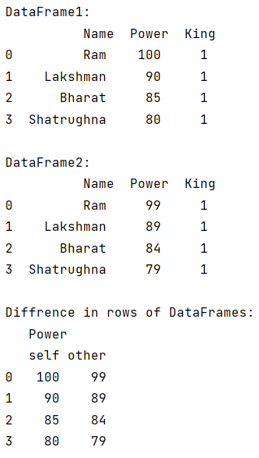# Python Pandas - Find difference between two dataframes

Given two Pandas DataFrames, we have to find the difference between them.
Submitted by Pranit Sharma, on August 07, 2022

Pandas is a special tool that allows us to perform complex manipulations of data effectively and efficiently. Inside pandas, we mostly deal with a dataset in the form of DataFrame. DataFrames are 2-dimensional data structures in pandas. DataFrames consist of rows, columns, and data.

Sometimes we deal with multiple DataFrames which can be almost similar with very slight changes, in that case, we might need to observe the differences between the DataFrames.

To find the difference between two DataFrames, we will check both the DataFrames if they are equal or not. To check if the DataFrames are equal or not, we will use pandas.DataFrame.compare() method.

Why do we need to compare two DataFrames? If we have multiple DataFrames with almost similar values then we are responsible for data ambiguity. The thumb rule is if you have two Datasets having identical data, keep all your data in one data set, you may have to add two or more extra columns to fit the remaining data but this will save a lot of time and space and helps in better understanding of data.

Let us understand with the help of an example,

## Python code to find difference between two dataframes

```# Importing pandas package
import pandas as pd

# Creating two dictionary
d1 = {
'Name':['Ram','Lakshman','Bharat','Shatrughna'],
'Power':[100,90,85,80],
'King':[1,1,1,1]
}

d2 = {
'Name':['Ram','Lakshman','Bharat','Shatrughna'],
'Power':[99,89,84,79],
'King':[1,1,1,1]
}

# Creating two separate DataFrames
df1 = pd.DataFrame(d1)
df2 = pd.DataFrame(d2)

# Display DataFrames
print("DataFrame1:\n",df1,"\n")
print("DataFrame2:\n",df2,"\n")

# Comparing the two DataFrames
check = df1.compare(df2)

# Display check
print("Differences in rows of DataFrames:\n",check)
```

Output: